/

### Standard Statistical Tests for Normality: The Cramér-von Mises test

The Cramér-von Mises test tests the null hypothesis that a sample,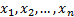comes from a pre-specified population distribution (or a pre-specified family of such distributions).

In its basic form, the test assumes that there are no parameters to be estimated for the distribution being tested, in which case the test and its set of critical values are distribution-free.

However, it is most commonly used where a family of distributions are being tested. For example, we might be testing whether the sample comes from a Normal distribution but without specifying in advance the mean and standard deviation of that distribution. It then becomes necessary to estimate the parameters on which the particular distribution depends and this needs to be taken into account by adjusting the test statistic and/or its critical values.

In its basic form, it involves the following test statistic,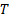, where we are testing the null hypothesis that the data is coming from a distribution with cumulative distribution function (cdf)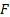: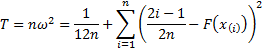where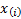is the’th order statistic, i.e. the’th smallest value in the sample. If the empirical distribution function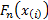is defined as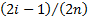then the statistic can be seen to be (up to a constant for any given) similar to the statistic used in the Kolmogorov-Smirnov test, but using the mean squared deviation rather than the supremum of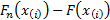.

Essentially the same approach can be used when testing whether data comes from a pre-specified family of distributions. However, the statistic must then be compared against critical values appropriate to the family in question and dependent also on the method used for parameter estimation.

Like the Kolmogorov-Smirnov test, the test can also in principle be inverted to give confidence limits on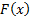itself and a variant can be used to test whether two (or more) underlying one-dimensional distributions differ. Generalising the statistic to more than one dimension is possible but complicated.